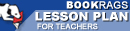Lesson Plans

# The Logic of Scientific Discovery Quiz | Four Week Quiz B

This set of Lesson Plans consists of approximately 102 pages of tests, essay questions, lessons, and other teaching materials.
 View a FREE sampleName: _________________________ Period: ___________________

This quiz consists of 5 multiple choice and 5 short answer questions through Part II: Chapter 9, Some Observations on Quantum Theory.

## Multiple Choice Questions

1. What does Popper consider existential statements to be?
(a) Tautological.
(b) Metaphysical.
(c) Logical.
(d) Concrete.

2. What does the first rule of logic do?
(a) States testability.
(b) Sets a norm.
(c) Determines the type of research.
(d) Proves a scientific statement.

3. What theory is based on random events?
(a) Random Theory.
(b) Theory of chance.
(c) Subjective theory.
(d) Normative theory.

4. What is Feigl's reasoning to the motion of simplicity?
(a) It reveals more empirical content.
(b) It can be better tested.
(c) It can be better justified.
(d) It appeals to the economy of thought.

5. What do the fractions between 0 and 1 represent?
(a) Tautological methods.
(b) Metaphysical numbers.
(c) Falsifiability.
(d) Justification.

1. What confuses the distinction between universal and individual names?

2. What method does Popper say applies to science and is important to the theory of knowledge?

3. What type of reasoning does Popper believe inductive reasoning is?

4. What increases with the amount of falsifiability?

5. What should the rules of epistemology adhere to?

 This section contains 186 words (approx. 1 page at 300 words per page) View a FREE sample